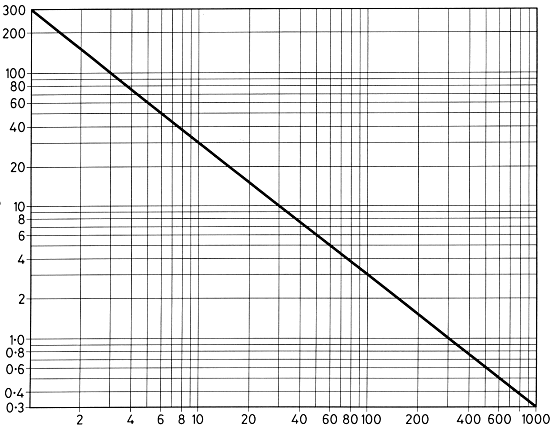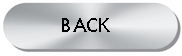# Frequency-Wavelength Conversion Graph

Some radios have their dials calibrated in metres (meters) and others in kilo Hertz (kHz) Mega Hertz (MHz). One kilo Hertz = one thousand cycles per second (kc/s) and one mega Mertz = one million cycles per second (Mc/s).

The product of the frequency and the wavelength of a radio signal is equal to the velocity of propagation of wireless waves which is the same as the velocity of light. Numerically: metres X Kilohertz = 300,000 (good enough)

To save you getting out a calculator print the graph below and keep it somewhere handy.The graph can be used to convert metres to MHz and vice versa. For example 400kHz (0.4MHz) = 750 metres using the vertical scale for frequency and reading off the horizontal scale for the wave length. The graph can be used the other way round as well, for example by using either the vertical or horizontal scale you will see that 100 metres = 3MHz.